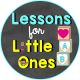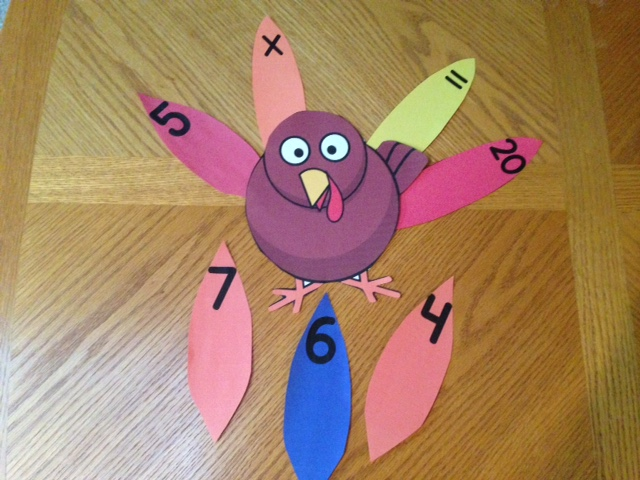# Thanksgiving Turkey Counting, Number Sequencing, Math Learning Center (Common Core Aligned)Posted byThis Thanksgiving Turkey Math Learning Center includes the following in both a color version AND a black and white version:

– turkey
– turkey feathers for numbers 0 – 20
– turkey feathers with dots for numbers 1-20 (subitizing)
– turkey feathers with tally marks for numbers 1-20
– turkey feathers for the following math symbols: +, -, x, division symbol, =, <, >
– blank turkey feathers that can be customized to your needs
– 4 student response sheets

Use this learning center to practice counting, number sense, number recognition, number sequencing, addition, subtraction, multiplication, division, greater than, less than, color recognition, to decorate Thanksgiving bulletin boards, and more. Blank feathers are included which allow you to customize the center to your students’ needs.

View sample pages

Practice number sense by writing a number on each turkey. Students must put the correct number feather, dot feather, and tally mark feather on each turkey or leave the turkeys blank and require students to sort the feathers.Use it to assess or practice common core state standard K.CC.A.2: Count forward beginning from a given number within the known sequence (instead of having to begin at 1). Simply place the beginning number of your choice’s feather in the turkey’s tail on the left side. The student must sequence the next 5 numbers by choosing the correct numbered feathers. The beginning number can be changed again and again.Assess and/or practice common core standards K.CC.B.4 & K.CC.B.5 (count to tell the number of objects). Place feathers on the turkey’s tail and have the student count the feathers and tell you how many or choose the correct number from a group of numbers or a group of the numbered feathers. Option 2: Place numbers on the turkeys and have students place the correct number of feathers on each turkey.

Assess and/or practice patterns by placing a few feathers on a turkey’s tail in a pattern. Have the student continue the pattern or do the same pattern on a turkey of their own.Use the greater than, less than feathers to practice common core state standard K.CC.C.7 Compare two numbers between 1 and 10 presented as written numerals. Place 2 numbered feathers on the turkey’s tail with a space in between them. Have the student place the greater than or less than feathers in between the 2 numbers.

The following kindergarten common core standards can be practiced with this center as well:
• CCSS.Math.Content.K.OA.A.4 For any number from 1 to 9, find the number that makes 10 when added to the given number, e.g., by using objects or drawings, and record the answer with a drawing or equation.
• CCSS.Math.Content.K.OA.A.5 Fluently add and subtract within 5.

Practice addition, subtraction, multiplication, and division by creating equations on the turkey’s tail leaving out a number which the student must supply. For example, 2 + 1 = , 8 – = 6, x 2 = 10, etc.The following common core state standards for grade 1 and up can also be practiced using this learning center:
• CCSS.Math.Content.1.OA.D.8 Determine the unknown whole number in an addition or subtraction equation relating three whole numbers. For example, determine the unknown number that makes the equation true in each of the equations 8 + ? = 11, 5 = _ – 3, 6 + 6 = _.
• CCSS.Math.Content.1.OA.D.7 Understand the meaning of the equal sign, and determine if equations involving addition and subtraction are true or false. For example, which of the following equations are true and which are false? 6 = 6, 7 = 8 – 1, 5 + 2 = 2 + 5, 4 + 1 = 5 + 2.
• CCSS.Math.Content.1.NBT.B.3 Compare two two-digit numbers based on meanings of the tens and ones digits, recording the results of comparisons with the symbols >, =, and <.
• CCSS.Math.Content.3.OA.A.4 Determine the unknown whole number in a multiplication or division equation relating three whole numbers. For example, determine the unknown number that makes the equation true in each of the equations 8 × ? = 48, 5 = _ ÷ 3, 6 × 6 = ?

# BUY NOW FOR ONLY \$2

This site uses Akismet to reduce spam. Learn how your comment data is processed.